# The Boron & De Weer Model of Intracellular pH Regulation

Rossana OcchipintiSoroush SafaeiPeter J. HunterWalter F. Boron
Abstract

The classic Boron & De Weer (1976) paper provided the first evidence of active regulation of $\mathrm{pH}$ in cells by an energy-dependent acid-base transporter. These authors also developed a quantitative model — comprising passive fluxes of acid-base equivalents across the cell membrane, intracellular reactions, and an active transport mechanism in the cell membrane (modelled as a proton pump) — to help interpret their measurements of intracellular $\mathrm{pH}$ under perturbations of both extracellular $\mathrm{CO_2}/\mathrm{HCO_3^-}$ and extracellular $\mathrm{NH_3}/\mathrm{NH_4^+}$. This Physiome paper seeks to make that model, and the experimental conditions under which it was developed, available in a reproducible and well-documented form, along with a software implementation that makes the model easy to use and understand. We have also taken the opportunity to update some of the units used in the original paper, and to provide a few parameter values that were missing in the original paper. Finally, we provide an historical background to the Boron & De Weer (1976) proposal for active $\mathrm{pH}$ regulation and a commentary on subsequent work that has enriched our understanding of this most basic aspect of cellular physiology.

Keywords:CO₂NH₃squid giant axonweak acidweak base

## 1Introduction¶

In 1976 Boron & De Weer published their landmark paper on “Intracellular $\mathrm{pH}$ transients in squid giant axons caused by $\mathrm{CO_2}$, $\mathrm{NH_3}$, and metabolic inhibitors” Boron & De Weer, 1976. The authors used a squid giant axon preparation and a mathematical model of $\mathrm{pH}$ buffering and the transport of protons, bicarbonate ($\mathrm{HCO_3^-}$) and $\mathrm{CO_2}$ to establish the experimental evidence for active regulation of intracellular $\mathrm{pH}$ ($\mathrm{pH_i}$) by a transporter in the plasma membrane that — at the expense of energy — either moves acid out of the cell, or base into the cell. Today, we refer to such a transporter generically as an acid-extrusion mechanism. For simplicity, Boron & De Weer modelled it as a proton pump, although the result would have been almost indistinguishable had they modelled it as the uptake of $\mathrm{HCO_3^-}$ or carbonate ($\mathrm{CO_3^{2-}}$). The paper reported on the consequences of adding and then removing extracellular $\mathrm{CO_2}/\mathrm{HCO_3^-}$, $\mathrm{NH_3/NH_4^+}$ (where $\mathrm{NH_4^+}$ is ammonium), or the metabolic inhibitors, cyanide, azide and dinitrophenol (DNP).

In the first experiment, following exposure of the cell to elevated $\mathrm{CO_2}$ and $\mathrm{HCO_3^-}$, $\mathrm{CO_2}$ rapidly enters the cell and intracellular $\mathrm{CO_2}$ equilibrates with the extracellular $\mathrm{CO_2}$, and generates intracellular $\mathrm{H^+}$ and $\mathrm{HCO_3^-}$ via the $\mathrm{CO_2}$ hydration reaction ($\mathrm{CO_2}+\mathrm{H_2O} \longrightarrow \mathrm{H^+}+\mathrm{HCO^-_3}$). The accumulating $\mathrm{H^+}$ results in a rapid fall of $\mathrm{pH_i}$ (see Figure 1A & Figure 1B). To the extent that the membrane is permeable to $\mathrm{HCO_3^-}$ as well as to $\mathrm{CO_2}$, $\mathrm{HCO_3^-}$ will initially enter the cell passively, along its electrochemical gradient. Soon, however, the accumulation of intracellular $\mathrm{HCO_3^-}$ reverses the $\mathrm{HCO_3^-}$ electrochemical gradient and would be expected to lead to the passive efflux of $\mathrm{HCO_3^-}$. This loss of cellular $\mathrm{HCO_3^-}$ would tend to acidify the cell because — to replenish the lost $\mathrm{HCO_3^-}$ — additional $\mathrm{CO_2}$ would enter the cell and form even more $\mathrm{H^+}$ and $\mathrm{HCO_3^-}$ (the passive $\mathrm{CO_2}/\mathrm{HCO_3^-}$ shuttle). Thus, the expectation was that prolonged exposure to $\mathrm{CO_2}$ would cause $\mathrm{pH_i}$ to fall rapidly (passive influx of $\mathrm{CO_2}$) and then to drift more slowly in the acidic direction (passive efflux of $\mathrm{HCO_3^-}$). In fact, Boron & De Weer observed an alkaline drift, leading to the postulate of active extrusion of $\mathrm{H^+}$ — or an equivalent process — at a rate that exceeds the passive shuttling by the $\mathrm{CO_2}/\mathrm{HCO_3^-}$ couple (see Figure 1A & Figure 1C).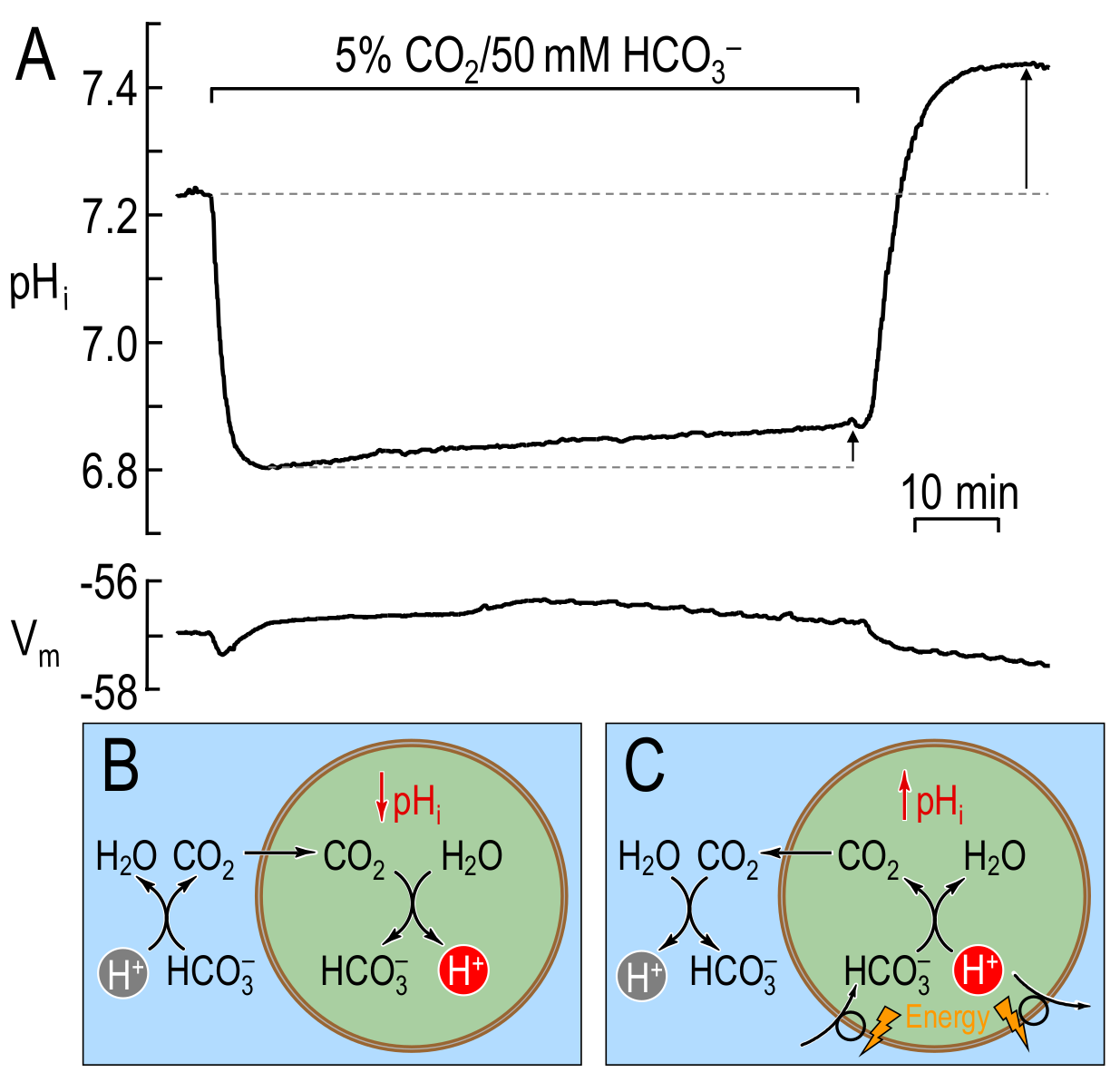Figure 1:pHi\mathrm{pH_i}pHi​ changes caused by prolonged exposure of a squid giant axon to extracellular CO2/HCO3−\mathrm{CO_2}/\mathrm{HCO_3^-}CO2​/HCO3−​ in the bulk solution. (A) Original pHi\mathrm{pH_i}pHi​ and VmV_\mathrm{m}Vm​ traces from figure 1 of BDW. Exposing an axon to extracellular CO2/HCO3−\mathrm{CO_2}/\mathrm{HCO_3^-}CO2​/HCO3−​ causes a rapid fall in pHi\mathrm{pH_i}pHi​ followed by a slow and sustained plateau-phase pHi\mathrm{pH_i}pHi​ recovery (i.e., pHi\mathrm{pH_i}pHi​ rises). Removing extracellular CO2/HCO3−\mathrm{CO_2}/\mathrm{HCO_3^-}CO2​/HCO3−​ causes pHi\mathrm{pH_i}pHi​ to overshoot its initial resting value. Both the plateau-phase recovery (short arrow) and the overshoot (long arrow) are indicative of net acid extrusion during the period of CO2/HCO3−\mathrm{CO_2}/\mathrm{HCO_3^-}CO2​/HCO3−​ exposure. (B) Cartoon illustrating the processes underlying the initial, rapid acidification phase in (A). The entry of CO2\mathrm{CO_2}CO2​ leads to the intracellular production of H+\mathrm{H^+}H+ (and thus to the observed pHi\mathrm{pH_i}pHi​ decay) via the reaction CO2+H2O⟶H++HCO3−\mathrm{CO_2}+\mathrm{H_2O} \longrightarrow \mathrm{H^+}+\mathrm{HCO^-_3}CO2​+H2​O⟶H++HCO3−​. (C) Cartoon illustrating the processes underlying the plateau-phase alkalinisation in (A). After CO2\mathrm{CO_2}CO2​ equilibration across the plasma membrane (pHi\mathrm{pH_i}pHi​ nadir in panel (A)), the slow entry of HCO3−\mathrm{HCO_3^-}HCO3−​ (or, equivalently, the slow exit of H+\mathrm{H^+}H+) — which has always been present but was overwhelmed by the influx of CO2\mathrm{CO_2}CO2​ — leads to the consumption of H+\mathrm{H^+}H+ (and thus to the observed slow pHi\mathrm{pH_i}pHi​ rise) via the reaction H++HCO3−⟶CO2+H2O\mathrm{H^+}+\mathrm{HCO^-_3} \longrightarrow \mathrm{CO_2}+\mathrm{H_2O}H++HCO3−​⟶CO2​+H2​O. The newly formed CO2\mathrm{CO_2}CO2​ then exits the cell. The observed pHi\mathrm{pH_i}pHi​ overshoot is the result of the accumulation of HCO3−\mathrm{HCO_3^-}HCO3−​ during exposure to extracellular CO2/HCO3−\mathrm{CO_2}/\mathrm{HCO_3^-}CO2​/HCO3−​. BDW used the mathematical model to postulate the presence of an active acid-extrusion mechanism that would explain both the observed plateau-phase pHi\mathrm{pH_i}pHi​ recovery and the pHi\mathrm{pH_i}pHi​ overshoot. (A), modified from Boron & De Weer (1976). (B)-(C), modified from Boron (2010).

Following removal of external $\mathrm{CO_2}$, intracellular $\mathrm{CO_2}$ diffuses out, while intracellular $\mathrm{HCO_3^-}$ combines with $\mathrm{H^+}$ to leave the cell as $\mathrm{CO_2}$. Thus, the entire intracellular $\mathrm{H^+}$ load associated with $\mathrm{CO_2}$ entry would be removed, returning $\mathrm{pH_i}$ to its value before the addition of $\mathrm{CO_2}/\mathrm{HCO_3^-}$ (or to a slightly lower value, to the extent that $\mathrm{HCO_3^-}$ had passively exited during the $\mathrm{CO_2}/\mathrm{HCO_3^-}$ exposure). In fact, Boron & De Weer observed that $\mathrm{pH_i}$ overshoots its resting value by an amount consistent with the net removal of $\mathrm{H^+}$ by the active, acid-extrusion mechanism during the $\mathrm{CO_2}/\mathrm{HCO_3^-}$ exposure (see Figure 1A & Figure 1C).

In the second experiment, following exposure of the cell to extracellular $\mathrm{NH_3}/\mathrm{NH_4^+}$ in the form of $\mathrm{NH_4Cl}$ (ammonium chloride), the intracellular environment rapidly becomes alkaline as $\mathrm{NH_3}$ enters and combines with $\mathrm{H^+}$ to form $\mathrm{NH_4^+}$ (equivalent to the hydration of $\mathrm{NH_3}$ to form $\mathrm{NH_4^+}$ and $\mathrm{OH^-}$). If this were the entire story, then $\mathrm{pH_i}$ would rise monotonically to a relatively alkaline value, and then the subsequent removal of $\mathrm{NH_4Cl}$ would cause $\mathrm{pH_i}$ to fall to precisely its initial value. In fact, Boron & De Weer observed that the exposure to $\mathrm{NH_4Cl}$ causes $\mathrm{pH_i}$ to rise rapidly and then fall slowly. Moreover, the subsequent removal of $\mathrm{NH_3}/\mathrm{NH_4^+}$ causes $\mathrm{pH_i}$ to undershoot its original value (see Figure 2A). Thus, Boron & De Weer postulated that, during the $\mathrm{NH_3}/\mathrm{NH_4^+}$ exposure, $\mathrm{NH_4^+}$ passively enters the cell down its electrochemical gradient. Early during the exposure, this $\mathrm{NH_4^+}$ influx would oppose the $\mathrm{NH_3}$ entry and slightly reduce the $\mathrm{pH_i}$ increase. Later during the $\mathrm{NH_4Cl}$ exposure, after intracellular $\mathrm{[NH_3]}$ rises to match extracellular $\mathrm{[NH_3]}$ ($\mathrm{[NH_3]_o}$), the continued passive influx of $\mathrm{NH_4^+}$ would generate intracellular $\mathrm{H^+}$ and $\mathrm{NH_3}$. The result would be a slow fall of $\mathrm{pH_i}$ and a rise in intracellular $\mathrm{[NH_3]}$, the latter leading to the passive exit of $\mathrm{NH_3}$ (the passive $\mathrm{NH_3}/\mathrm{NH_4^+}$ shuttle, see Figure 2A & Figure 2B). The experimental data are consistent with the proposed mathematical model.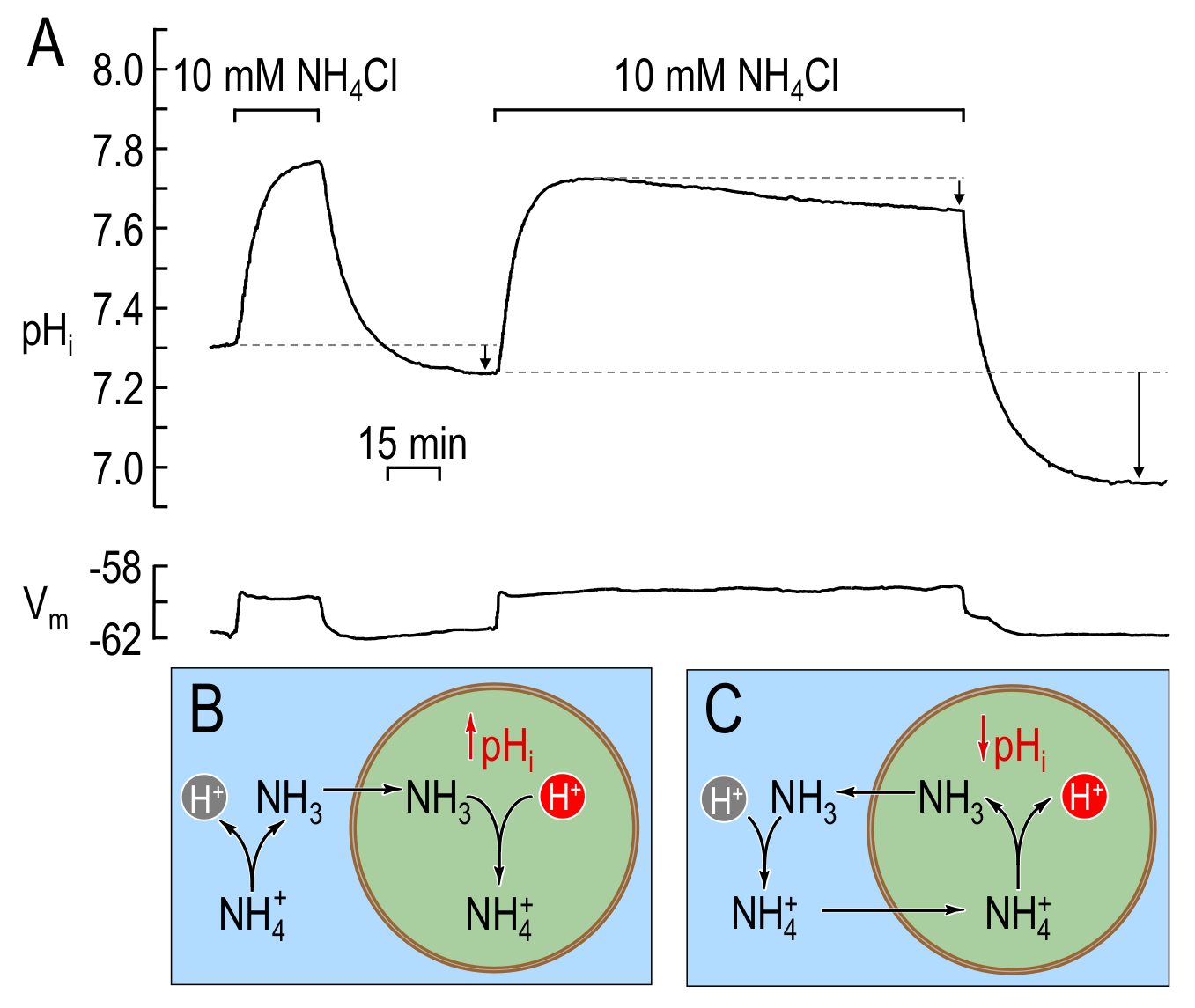Figure 2:pHi\mathrm{pH_i}pHi​ changes caused by a short and a long exposure of a squid giant axon to extracellular NH3/NH4+\mathrm{NH_3}/\mathrm{NH_4^+}NH3​/NH4+​ in the bulk solution. (A) Original pHi\mathrm{pH_i}pHi​ and VmV_\mathrm{m}Vm​ traces from figure 2 of BDW. A short exposure of the axon to extracellular NH3/NH4+\mathrm{NH_3}/\mathrm{NH_4^+}NH3​/NH4+​ causes a rapid rise in pHi\mathrm{pH_i}pHi​, followed by a pHi\mathrm{pH_i}pHi​ decay that modestly undershoots (lower short arrow) its initial resting value upon removal of extracellular NH3/NH4+\mathrm{NH_3}/\mathrm{NH_4^+}NH3​/NH4+​. A longer exposure of squid giant axons to extracellular NH3/NH4+\mathrm{NH_3}/\mathrm{NH_4^+}NH3​/NH4+​ causes a rapid rise in pHi\mathrm{pH_i}pHi​, followed by a slow and sustained pHi\mathrm{pH_i}pHi​ decay. Removing extracellular NH3/NH4+\mathrm{NH_3}/\mathrm{NH_4^+}NH3​/NH4+​ causes pHi\mathrm{pH_i}pHi​ to undershoot substantially its initial resting value (long arrow). Both the plateau-phase acidification (upper short arrow) and the undershoot (long arrow) are indicative of net acid loading during the period of NH3/NH4+\mathrm{NH_3}/\mathrm{NH_4^+}NH3​/NH4+​ exposure. (B) Cartoon illustrating the processes underlying the initial alkalinisation phase in (A) for both short and long exposures to extracellular NH3/NH4+\mathrm{NH_3}/\mathrm{NH_4^+}NH3​/NH4+​. The initial entry of NH3\mathrm{NH_3}NH3​ leads to the intracellular consumption of H+\mathrm{H^+}H+ (and thus to the observed pHi\mathrm{pH_i}pHi​ rise) via the reaction NH3+H+⟶NH4+\mathrm{NH_3}+\mathrm{H^+} \longrightarrow \mathrm{NH^+_4}NH3​+H+⟶NH4+​. (C) Cartoon illustrating the processes underlying the plateau-phase acidification during the long NH3/NH4+\mathrm{NH_3}/\mathrm{NH_4^+}NH3​/NH4+​ exposure in (A). After NH3\mathrm{NH_3}NH3​ equilibration across the plasma membrane (pHi\mathrm{pH_i}pHi​ peak in panel (A)), the slow entry of NH4+\mathrm{NH_4^+}NH4+​ — which has always been present but overwhelmed by the influx of NH3\mathrm{NH_3}NH3​ — leads to the production of H+\mathrm{H^+}H+ (and thus to the observed slow pHi\mathrm{pH_i}pHi​ decay during the plateau phase) via the reaction NH4+⟶NH3+H+\mathrm{NH^+_4} \longrightarrow \mathrm{NH_3}+\mathrm{H^+}NH4+​⟶NH3​+H+. The newly formed NH3\mathrm{NH_3}NH3​ then exits the cell. The pHi\mathrm{pH_i}pHi​ undershoots observed upon removal of extracellular NH3/NH4+\mathrm{NH_3}/\mathrm{NH_4^+}NH3​/NH4+​, during both short and long NH3/NH4+\mathrm{NH_3}/\mathrm{NH_4^+}NH3​/NH4+​ exposures, are the result of the accumulation of NH4+\mathrm{NH_4^+}NH4+​ during exposure to extracellular NH3/NH4+\mathrm{NH_3}/\mathrm{NH_4^+}NH3​/NH4+​. BDW used the mathematical model to postulate the above sequence of events, including both the plateau-phase acidification and the pHi\mathrm{pH_i}pHi​ undershoot. (A), modified from Boron & De Weer (1976). (B)-(C), modified from Boron (2010).

Finally, exposure of the cells — in turn — to cyanide, DNP and azide resulted in intracellular acidosis, consistent with the accumulation of acid metabolites.

In the present paper, we re-formulate the models from Boron & De Weer (1976), henceforth referred to as ‘BDW’, and specify the simulation using the Physiome modelling standards CellML Cuellar et al., 2003 and SED-ML Bergmann et al., 2017 in order to ensure that the model reproduces the graphs in the original paper and that the model is fully curated. Note that this effort requires the specification of some parameters used in BDW’s simulations, but not described in the BDW paper. The curated and annotated model is made available in a form that users can run with OpenCOR to understand the model and to explore the effect of changes in parameter values.

## 2pH Buffering by Weak Acids and Weak Bases¶

We begin by reviewing a few rudimentary concepts of $\mathrm{pH}$ buffering by weak acids and bases Roos & Boron, 1981Bevensee & Boron, 2013Boron & Boulpaep, 2016 to provide the background for understanding the derivation and implementation of the BDW model.

##### Buffers.¶

According to Brönsted’s definition Brönsted, 1923, an acid is any substance that can donate a $\mathrm{H^+}$. Conversely, a base is any substance that can accept a $\mathrm{H^+}$. A buffer is any substance that can reversibly consume or produce $\mathrm{H^+}$, thereby minimising changes in $\mathrm{pH}$.

The dissociation of the uncharged weak acid ($\mathrm{HA}$) to the anionic weak base ($\mathrm{A^-}$) is described by the equilibrium reaction:

$\mathrm{HA \rightleftharpoons A^- + H^+}$

which is governed by the equilibrium constant

$K_\mathrm{HA}=\dfrac{\mathrm{[A^-][H^+]}}{\mathrm{[HA]}}.$

An example is the carbonic acid ($\mathrm{H_2CO_3}$) dissociation reaction,

$\mathrm{H_2CO_3 \rightleftharpoons HCO_3^- + H^+}.$

The total weak acid concentration, $\mathrm{[TA]}$, is the sum of $\mathrm{[HA]}$ and $\mathrm{[A^-]}$. Note that $\mathrm{[TA]}$ is one of the two main unknowns in the BDW model for weak acids.

The dissociation of the cationic weak acid ($\mathrm{BH^+}$) to the uncharged weak base ($\mathrm{B}$) is described by the equilibrium reaction,

$\mathrm{BH^+ \rightleftharpoons B + H^+},$

where the equilibrium constant is

$K_\mathrm{BH}=\dfrac{\mathrm{[B][H^+]}}{\mathrm{[BH^+]}}.$

An example is the $\mathrm{NH_4^+}$ dissociation reaction,

$\mathrm{NH_4^+ \rightleftharpoons NH_3 + H^+}.$

The total weak base concentration, $\mathrm{[TB]}$, is the sum of $\mathrm{[BH^+]}$ and $\mathrm{[B]}$. Note that $\mathrm{[TB]}$ is one of the two main unknowns in the BDW model for weak bases.

##### The $\mathrm{CO_2}/\mathrm{HCO_3^-}$ buffer pair.¶

The formation of $\mathrm{HCO_3^-}$ and $\mathrm{H^+}$ from $\mathrm{CO_2}$ by hydration is given by the equilibrium reaction

$\mathrm{CO_2 + H_2O \rightleftharpoons HCO_3^- + H^+},$

where the equilibrium constant is

$K_\mathrm{CO_2}=\dfrac{\mathrm{[HCO_3^-][H^+]}}{\mathrm{[CO_2]}}.$

Taking logarithms of both sides of (5), and recognising from Henry’s law that

$\mathrm{[CO_2]}=s\cdot p_\mathrm{CO_2},$

where $s$ is the solubility coefficient for $\mathrm{CO_2}$ and $p_\mathrm{CO_2}$ is the partial pressure of $\mathrm{CO_2}$, we obtain the familiar Henderson-Hasselbalch equation

$\mathrm{pH}=\mathrm{pK_{CO_2}}+\log{\dfrac{\mathrm{[HCO_3^-]}}{s\cdot p_\mathrm{CO_2}}}.$

Here, $\mathrm{pH}=-\log[\mathrm{H^+}]$ and $\mathrm{pK_{CO_2}}=-\log(K_\mathrm{CO_2})$.

In terms of the nomenclature above, one might regard $\mathrm{CO_2}$ as the weak acid $\mathrm{HA}$, and $\mathrm{HCO_3^-}$ as its conjugate base $\mathrm{A^-}$.

##### Buffering power ($\beta$).¶

By definition, $\beta$ is the amount of strong base (e.g., $\mathrm{NaOH}$), or the negative of the amount of strong acid (e.g., $\mathrm{HCl}$), that one must add to $1~\mathrm{L}$ of solution to raise $\mathrm{pH}$ by one $\mathrm{pH}$ unit:

$\beta=\dfrac{\Delta\text{[Strong~Base]}}{\Delta \mathrm{pH}}=-\dfrac{\Delta\text{[Strong~Acid]}}{\Delta \mathrm{pH}}.$

The units of $\beta$ are $\mathrm{mM}$. For additional details, refer to Roos & Boron (1981)Boron & Boulpaep (2016). Note that BDW defined $\beta$ as a negative number, as did Koppel and Spiro in their original definition of buffering Koppel, 1914Roos & Boron, 1980, rather than as a now-conventional positive quantity, as did Van Slyke in his later work Van Slyke, 1922. BDW’s definition, which they consistently applied, has no effect on the outcome of their simulations. In the present paper, we will follow the definition of Van Slyke — defining $\beta$ as a positive number — and make appropriate sign changes to the derived equations.

## 3The Boron & De Weer Model for the Permeation by an Uncharged Weak Acid and its Conjugate, Anionic Weak Base¶

The BDW mathematical model consists of two time-dependent ordinary differential equations (ODEs), one describing the time-course of the concentration of total intracellular buffer ($\mathrm{[TA]_i} = \mathrm{[HA]_i}+\mathrm{[A^-]_i}$) and the other the time-course of the intracellular free $\mathrm{H^+}$ concentration (which is related to $\mathrm{pH_i}$). BDW derived these two equations for the general cases in which any buffer pair $\mathrm{HA}/\mathrm{A^-}$, or any buffer pair $\mathrm{B}/\mathrm{BH^+}$, can move passively across the plasma membrane of a prototype cell. Then, they applied these two general equations to their specific experimental conditions, namely exposure of a cell (a squid giant axon) to equilibrated extracellular $\mathrm{CO_2}/\mathrm{HCO_3^-}$ or to equilibrated extracellular $\mathrm{NH_3}/\mathrm{NH_4^+}$.

Here, following BDW’s approach, we begin by deriving the equations for $\mathrm{HA}/\mathrm{A^-}$. In the next section, we apply the same general formalism to $\mathrm{B}/\mathrm{BH^+}$.

##### Derivation for weak acids.¶

Imagine that a cell is exposed to a solution containing equilibrated $\mathrm{HA}/\mathrm{A^-}$ and that both $\mathrm{HA}$ and $\mathrm{A^-}$ initially move into the cell — because of the chemical gradient in the case of $\mathrm{HA}$, and because of the electrochemical gradient in the case of $\mathrm{A^-}$.

An integrated form of Fick’s first law of diffusion describes the net passive influx of $\mathrm{HA}$ ($J_\mathrm{HA}$)

$J_\mathrm{HA}=P_\mathrm{HA}\bigg(\mathrm{[HA]_o}-\mathrm{[HA]_i}\bigg),$

where $J_\mathrm{HA}$ is flux ($\mathrm{mol\cdot m^{-2}\cdot s^{-1}}$) and $P_\mathrm{HA}$ ($\mathrm{m\cdot s^{-1}}$) is the membrane permeability to the uncharged weak acid $\mathrm{HA}$. Note that this is a passive diffusion equation because $\mathrm{HA}$ is uncharged.

The constant field equation — also known as the Goldman, Hodgkin, Katz (GHK) Goldman, 1943Hodgkin & Huxley, 1952 equation — describes the net passive influx of $\mathrm{A^-}$ ($J_\mathrm{A^-}$):

$J_\mathrm{A^-}=P_\mathrm{A^-}\left(\dfrac{V_\mathrm{m}F}{RT}\right)\left(\dfrac{\mathrm{[A^-]_o}-\epsilon\mathrm{[A^-]_i}}{1-\epsilon}\right),$

where $P_\mathrm{A^-}$ ($\mathrm{m\cdot s^{-1}}$) is the membrane permeability to the charged conjugate base $\mathrm{A^-}$, $V_\mathrm{m}$ is the membrane potential (intracellular relative to extracellular potential), and $\epsilon$ is a shorthand for $e^{{-V_\mathrm{m}F}/{RT}}$. Note that $J_\mathrm{HA}$ and $J_\mathrm{A^-}$ have units of $\mathrm{mol\cdot m^{-2}\cdot s^{-1}}$.

Although $\mathrm{HA}$ and $\mathrm{A^-}$ can interconvert in the cytosol, BDW assumed that the intracellular concentration of total weak acid $\mathrm{[TA]_i}$ only can change due to the transmembrane fluxes of $\mathrm{HA}$ and $\mathrm{A^-}$ (see Figure 3). Thus, the time rate of change of $\mathrm{[TA]_i}$ is

$\dfrac{\mathrm{d[TA]_i}}{\mathrm{d}t}=\rho\left(J_\mathrm{HA}+J_\mathrm{A^-}\right),$

where $\rho$ ($\mathrm{m^{-1}}$) is the area-to-volume ratio for the cell, and converts the transmembrane flux per unit area (in units of $\mathrm{mol\cdot m^{-2}\cdot s^{-1}}$) to a time rate of change per unit cell volume ($\mathrm{mol\cdot m^{-3}\cdot s^{-1}}$ or $\mathrm{mM\cdot s^{-1}}$). (11) is the first of two ODEs of the BDW model for the buffer pair $\mathrm{HA}/\mathrm{A^-}$.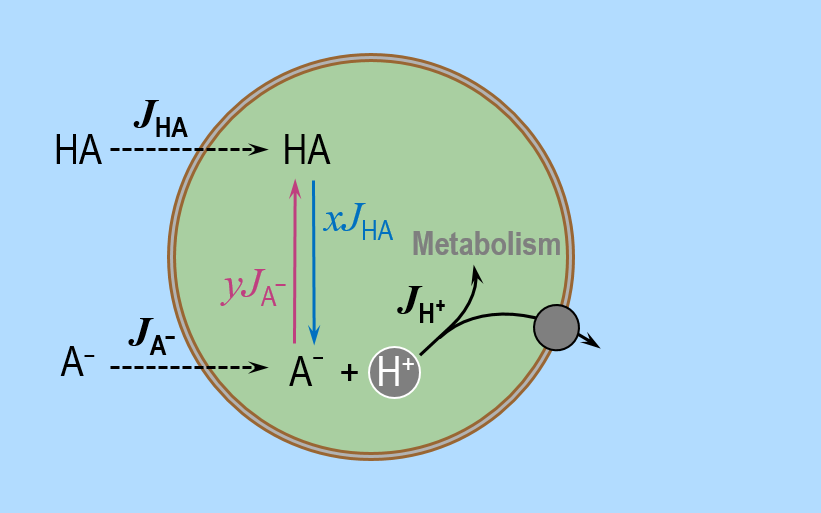Figure 3:Cartoon illustrating the main assumptions in the BDW model of permeating uncharged weak acid HA\mathrm{HA}HA and its conjugate anionic weak base A−\mathrm{A^-}A−. The BDW model consists of two time-dependent ODEs. The first one describes the time-course of the intracellular concentration of total weak acid [TA]i\mathrm{[TA]_i}[TA]i​, and the second one describes the time-course of [H+]i\mathrm{[H^+]_i}[H+]i​. BDW assumed that [TA]i\mathrm{[TA]_i}[TA]i​ changes in time because of the transmembrane fluxes of HA\mathrm{HA}HA (JHAJ_\mathrm{HA}JHA​) — modelled according to Fick’s first law of diffusion — and A−\mathrm{A^-}A− (JA−J_\mathrm{A^-}JA−​) — modelled according to the Goldman, Hodgkin, Katz (GHK) equation. According to BDW, the time rate of change of [H+]i\mathrm{[H^+]_i}[H+]i​ depends on the net rate dQ/dt\mathrm{d}Q/\mathrm{d}tdQ/dt at which acids are added into the cytosol. BDW assumed that dQ/dt\mathrm{d}Q/\mathrm{d}tdQ/dt depends on (i) the release of H+\mathrm{H^+}H+ by some fraction xxx of the entering HA\mathrm{HA}HA (i.e., xJHAxJ_\mathrm{HA}xJHA​), (ii) the consumption of H+\mathrm{H^+}H+ by some fraction yyy of the entering A−\mathrm{A^-}A− (i.e., yJA−yJ_\mathrm{A^-}yJA−​), and (iii) the additional rate of intracellular H+\mathrm{H^+}H+ consumption via metabolism or active acid extrusion (JH+J_\mathrm{H^+}JH+​).

Later, Bevensee and Boron defined the time rate of change per unit volume (e.g., $\mathrm{d[TA]_i/dt}$) as a ‘pseudoflux’ $\phi$, with the area-to-volume ratio folded into the value of $\phi$ Bevensee & Boron, 2013. Physiologists sometimes prefer to present experimental data in terms of pseudoflux because most mammalian cells often have complex geometries that make it difficult to estimate surface area.

In deriving the second ODE of their model, BDW started by noting that the time rate of change of free protons, $\mathrm{d[H^+]_i}/\mathrm{d}t$, depends on the rate at which acids are added into the cytosol per unit volume and per unit time — denoted $\mathrm{d}Q/\mathrm{d}t$ ($\mathrm{mol\cdot m^{-3}\cdot s^{-1}}$) where $Q$ is the total intracellular acid content. Like $\mathrm{d[TA]_i}/\mathrm{d}t$, both $\mathrm{d[H^+]_i}/\mathrm{d}t$ and $\mathrm{d}Q/\mathrm{d}t$ are pseudofluxes.

In their simple system of a squid giant axon exposed to $\mathrm{CO_2}$ (i.e., $\mathrm{HA}$) and $\mathrm{HCO_3^-}$ (i.e., $\mathrm{A^-}$), BDW assumed that only three general processes affect $\mathrm{d}Q/\mathrm{d}t$: (i) the release of $\mathrm{H^+}$ by some fraction ($x$) of the entering $\mathrm{HA}$ (i.e., $xJ_\mathrm{HA}$), (ii) the consumption of $\mathrm{H^+}$ by some fraction $(y)$ of the entering $\mathrm{A^-}$ (i.e., $yJ_\mathrm{A^-}$), and (iii) the “additional” rate of intracellular consumption or active extrusion of $\mathrm{H^+}$ ($J_\mathrm{H^+}$; see Figure 3) above the fixed background rate of $\mathrm{H^+}$ extrusion necessary to balance the fixed background rate of acid loading (i.e., addition of $\mathrm{H^+}$ or equivalent acid, or removal of $\mathrm{OH^-}$ or equivalent base) in the absence of $\mathrm{HA}/\mathrm{A^-}$. Thus,

$\dfrac{\mathrm{d}Q}{\mathrm{d}t}=\rho\left(xJ_\mathrm{HA}-yJ_\mathrm{A^-}-J_\mathrm{H^+}\right).$

A critical insight by BDW is that during each infinitesimal increment in time during which a bolus of $\mathrm{HA}$ enters the cell, the entering $\mathrm{HA}$ redistributes itself between $\mathrm{HA}$ (and $\mathrm{H^+}$) vs $\mathrm{A^-}$, according to the pre-existing ratios $\mathrm{[HA]_i}/\mathrm{[TA]_i}$ and $\mathrm{[A^-]_i}/\mathrm{[TA]_i}$. Thus, the fraction $y$ of entering $\mathrm{HA}$ that remains $\mathrm{HA}$ is

$y=\dfrac{\mathrm{[HA]_i}}{\mathrm{[TA]_i}}=\dfrac{\mathrm{[HA]_i}}{\mathrm{[HA]_i}+\mathrm{[A^-]_i}}.$

This is also the fraction of entering $\mathrm{A^-}$ that combines with $\mathrm{H^+}$ and becomes $\mathrm{HA}$. Combining the above expression with (2),

$y=\dfrac{\mathrm{[HA]_i}}{\mathrm{[HA]_i}+\mathrm{[A^-]_i}}=\dfrac{\mathrm{[H^+]_i}}{\mathrm{[H^+]_i}+K_\mathrm{HA}}=\alpha,$

which BDW defined as $\alpha$. Conversely, the fraction $x$ of $\mathrm{A^-}$ that remains $\mathrm{A^-}$ is

$x=\dfrac{\mathrm{[A^-]_i}}{\mathrm{[HA]_i}+\mathrm{[A^-]_i}}.$

This is also the fraction of entering $\mathrm{HA}$ that dissociates to form $\mathrm{A^-}$ and $\mathrm{H^+}$. Combining the above expression with (2),

$x=\dfrac{\mathrm{[A^-]_i}}{\mathrm{[HA]_i}+\mathrm{[A^-]_i}}=\dfrac{K_\mathrm{HA}}{\mathrm{[H^+]_i}+K_\mathrm{HA}}=1-\alpha.$

In summary, (12) becomes:

$\dfrac{\mathrm{d}Q}{\mathrm{d}t}=\rho\bigg((1-\alpha)J_\mathrm{HA}-\alpha J_\mathrm{A^-}-J_\mathrm{H^+}\bigg).$

BDW modelled $J_\mathrm{H^+}$ ($\mathrm{mol\cdot m^{-2}\cdot s^{-1}}$) as the additional proton-extrusion rate above the fixed background rate

$J_\mathrm{H^+}= \begin{cases} \dfrac{k}{\rho}\bigg(\mathrm{[H^+]_i}-\mathrm{[H^+]'_i}\bigg) & \mathrm{pH_i} < \mathrm{{pH}'_i}, \\ 0 & \mathrm{otherwise}, \end{cases}$

where $k$ ($\mathrm{s^{-1}}$) is the proton-pumping rate constant, $(k/\rho)\mathrm{[H^+]_i}$ is the additional flux of $\mathrm{H^+}$ above the background $\mathrm{H^+}$ flux of $(k/\rho)\mathrm{[H^+]'_i}$, which occurs at the resting $\mathrm{[H^+]_i}$ of $\mathrm{[H^+]'_i}$ (i.e., resting $\mathrm{pH_i}$ of $\mathrm{{pH}'_i}$). Note that $k/\rho$ has units of ($\mathrm{m\cdot s^{-1}}$), consistent with the membrane permeability terms $P_\mathrm{HA}$ and $P_\mathrm{A^-}$ in (9) and (10).

The BDW authors used the definition of buffering power, in its infinitesimal form, to derive the relation between $\mathrm{d}{\mathrm{[H^+]_i}}/\mathrm{d}t$ and $\mathrm{d}Q/\mathrm{d}t$, as shown in the following steps.

Our first goal is to obtain an expression for $\mathrm{d}{\mathrm{pH}}/\mathrm{d}t$ in terms of $\mathrm{d}Q/\mathrm{d}t$. According to the chain rule:

$\dfrac{\mathrm{dpH}}{\mathrm{d}t}=\left(\dfrac{\mathrm{dpH}}{\mathrm{d}Q}\right) \left(\dfrac{\mathrm{d}Q}{\mathrm{d}t}\right).$

By definition (see (8)), $\beta=-{\mathrm{d}Q}/{\mathrm{dpH}}$ ($\mathrm{mol\cdot m^{-3}}$), or equivalently

$\dfrac{\mathrm{dpH}}{\mathrm{d}Q}=-\dfrac{1}{\beta}.$

Combining (19) and (20)

$\dfrac{\mathrm{dpH}}{\mathrm{d}t}=\left(-\dfrac{1}{\beta}\right)\left(\dfrac{\mathrm{d}Q}{\mathrm{d}t}\right).$

Our next goal is to obtain an expression for $\dfrac{\mathrm{dpH}}{\mathrm{d}t}$ in terms of $\dfrac{\mathrm{d[H^+]_i}}{\mathrm{d}t}$. According to the chain rule:

$\dfrac{\mathrm{dpH}}{\mathrm{d}t}=\left(\dfrac{\mathrm{dpH}}{\mathrm{d[H^+]_i}}\right)\left( \dfrac{\mathrm{d[H^+]_i}}{\mathrm{d}t}\right).$

By definition, $\mathrm{pH}={-\ln{\mathrm{[H^+]_i}}}/{2.303}$, so that:

$\dfrac{\mathrm{dpH}}{\mathrm{d}{\mathrm{[H^+]_i}}}=-\dfrac{1}{2.303\mathrm{[H^+]_i}}.$

Combining (22) and (23), we have

$\dfrac{\mathrm{dpH}}{\mathrm{d}t}=\left(-\dfrac{1}{2.303\mathrm{[H^+]_i}}\right)\left(\dfrac{\mathrm{d}\mathrm{[H^+]_i}}{\mathrm{d}t}\right),$

or equivalently,

$\dfrac{\mathrm{d[H^+]_i}}{\mathrm{dt}}=-2.303\mathrm{[H^+]_i}\left(\dfrac{\mathrm{dpH}}{\mathrm{d}t}\right).$

Substituting (21) into (25), we obtain

$\dfrac{\mathrm{d[H^+]_i}}{\mathrm{dt}}=\left(\dfrac{2.303\mathrm{[H^+]_i}}{\beta}\right)\left(\dfrac{\mathrm{d}Q}{\mathrm{d}t}\right).$

Finally, substituting (17) into (26),

$\dfrac{\mathrm{d[H^+]_i}}{\mathrm{dt}}=\left(\dfrac{2.303\mathrm{[H^+]_i}}{\beta}\right) \rho\bigg((1-\alpha)J_\mathrm{HA}-\alpha J_\mathrm{A^-}-J_\mathrm{H^+}\bigg),$

which is the second equation of the BDW model.

Substituting for $\alpha$ (from (14)), $[\mathrm{HA]_i} = \alpha [\mathrm{TA]_i}, [\mathrm{A^-]_i} = (1-\alpha) [\mathrm{TA]_i}$, in (11) and (27), we obtain the two ODEs of the BDW model in terms of $[\mathrm{TA]_i}$ and $\mathrm{[H^+]_i}$:

$\dfrac{\mathrm{d[TA]_i}}{\mathrm{d}t}=\rho\left(J_\mathrm{HA}+J_\mathrm{A^-}\right),$
$\dfrac{\mathrm{d[H^+]_i}}{\mathrm{d}t}=\left(\dfrac{2.303\mathrm{[H^+]_i}}{\beta}\right)\rho\left(\left(\dfrac{K_\mathrm{HA}}{\mathrm{[H^+]_i}+K_\mathrm{HA}}\right)J_\mathrm{HA}-\left(\dfrac{\mathrm{[H^+]_i}}{\mathrm{[H^+]_i}+K_\mathrm{HA}}\right)J_\mathrm{A^-}-J_\mathrm{H^+} \right),$

where $J_\mathrm{HA}$ (from (9)), and $J_\mathrm{A^-}$ (from (10)) are given by:

$J_\mathrm{HA}=P_\mathrm{HA}\left( \mathrm{[HA]_o}-\dfrac{\mathrm{[H^+]_i}}{\mathrm{[H^+]_i}+K_\mathrm{{HA}}}\mathrm{[TA]_i} \right),$
$J_\mathrm{A^-}=P_\mathrm{A^-}\left(\dfrac{V_\mathrm{m}F}{RT}\right)\left(\dfrac{\mathrm{[A^-]_o}-\dfrac{K_\mathrm{HA}}{\mathrm{[H^+]_i}+K_\mathrm{HA}}\mathrm{[TA]_i} \epsilon}{1-\epsilon}\right),$

and $J_\mathrm{H^+}$ is given by (18).

The numerical solution of the above two equations yields the time courses of $[\mathrm{TA]_i}$ and $\mathrm{[H^+]_i}$, which in turn yield the time-courses of $[\mathrm{HA]_i}$ and $[\mathrm{A^-]_i}$ via:

$\mathrm{[HA]_i}=\alpha\mathrm{[TA]_i},$
$\mathrm{[A^-]_i}=(1-\alpha)\mathrm{[TA]_i},$

where

$\alpha=\dfrac{\mathrm{[H^+]_i}}{\mathrm{[H^+]_i}+K_\mathrm{HA}}.$
##### Simulation for $\mathrm{CO_2}/\mathrm{HCO_3^-}$ experiments.¶

BDW employed (28) and (29) to simulate the experiments in which they exposed a squid giant axon to a solution containing equilibrated $\mathrm{CO_2}/\mathrm{HCO_3^-}$. Their simulation protocol was a step change in (a) extracellular $p_\mathrm{CO_2}$ from $0$ to $5\%$ $\mathrm{CO_2}$ ($37$ $\mathrm{mmHg}$ or, with $s=0.0321$ $\mathrm{mM\cdot mmHg^{-1}}$, $\mathrm{[CO_2]_o}=s.p_\mathrm{CO_2}=1.1877~\mathrm{mM}$) and (b) extracellular $\mathrm{[HCO_3^-]}$ from $0$ to $59.5260$ $\mathrm{mM}$ (the value that $\mathrm{[HCO_3^-]_o}$ has in a solution containing $5\%$ $\mathrm{CO_2}$ at $\mathrm{pH_o}$ of $7.70$). The step change is applied for $2700$ $\mathrm{s}$ ($45~\mathrm{min}$) at constant $\mathrm{pH_o}=7.70$.

Table 1 and Table 2 report the parameter values used by BDW. Table 1 provides parameter values that are common to both the $\mathrm{CO_2}/\mathrm{HCO_3^-}$ and the $\mathrm{NH_3}/\mathrm{NH_4^+}$ experiments. Table 2 provides parameter values exclusive to the $\mathrm{CO_2}/\mathrm{HCO_3^-}$ experiments only.

In the present work, the differential (28) and (29) — when coded in CellML and solved with OpenCOR — produce the plots in Figure 4. The simulation file Boron-CO2.sedml contains the computational setting for running the model. Open the .sedml file in OpenCOR and click Run Simulation. The initial conditions are $[\mathrm{TA]_i}=0$ $\mathrm{mM}$ and $\mathrm{pH_i}=7.40$. Note that Figure 4 illustrates the time courses not only of $\mathrm{pH_i}$ — as presented by BDW — but also of quantities (e.g., various solute concentrations and fluxes) not displayed in the original paper; these values are useful for understanding the processes that contribute to the $\mathrm{pH_i}$ transient. Moreover, our curated and annotated version of the BDW model also allows one to alter the parameter values from those originally chosen by BDW, thereby extending the ability of the user to investigate the predictive power of the computational model.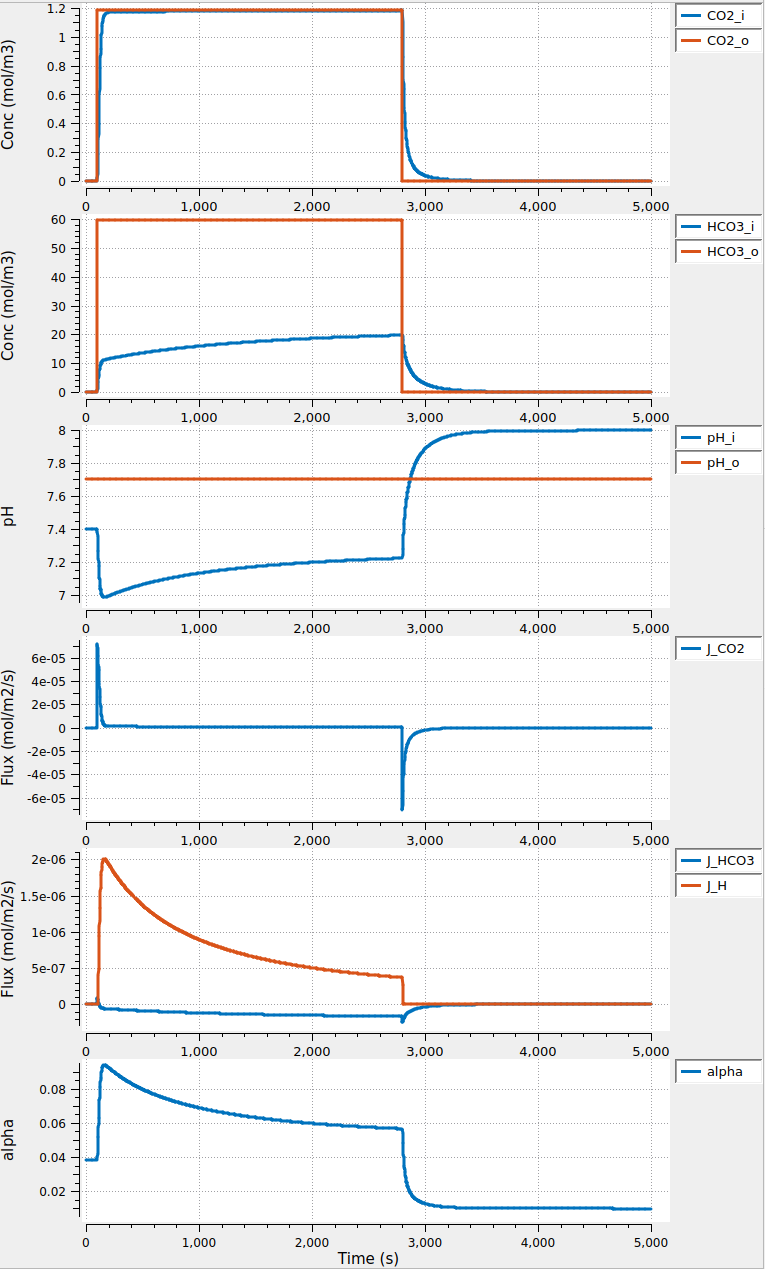Figure 4:Solution of the BDW model during and following a 2700 s2700~\mathrm{s}2700 s period of externally applied CO2\mathrm{CO_2}CO2​. In these simulations pHo=7.70\mathrm{pH_o}=7.70pHo​=7.70 and [HCO3−]o\mathrm{[HCO_3^-]_o}[HCO3−​]o​ is determined from the equilibrium with [H+]o\mathrm{[H^+]_o}[H+]o​ and CO2\mathrm{CO_2}CO2​ (footnote 9). Note that, during the plateau phase, [HCO3−]i\mathrm{[HCO_3^-]_i}[HCO3−​]i​ continues to rise as pHi\mathrm{pH_i}pHi​ rises at a constant [CO2]i\mathrm{[CO_2]_i}[CO2​]i​ (the proton pumping rate kkk is set to 300300300 s−1\mathrm{s^{-1}}s−1, thus k/ρ=0.0375k/\rho= 0.0375k/ρ=0.0375 m⋅s−1\mathrm{m\cdot s^{-1}}m⋅s−1). Note also that, after the removal of CO2/HCO3−\mathrm{CO_2}/\mathrm{HCO_3^-}CO2​/HCO3−​, pHi\mathrm{pH_i}pHi​ rises to a higher value (∼8.15\sim 8.15∼8.15) than its starting value (∼7.4\sim 7.4∼7.4), indicating the net extrusion of acid from the cell during the CO2/HCO3−\mathrm{CO_2}/\mathrm{HCO_3^-}CO2​/HCO3−​ exposure.

## 4The Boron & De Weer Model for the Permeation by an Uncharged Weak Base and its Conjugate, Cationic Weak Acid¶

Following an approach analogous to the one outlined above for weak acids, BDW derived two time-dependent ODEs. The first describes the time-course of the concentration of total intracellular buffer ($[\mathrm{TB]_i} = [\mathrm{B]_i}+[\mathrm{BH^+]_i}$), and the other the time-course of the intracellular free $\mathrm{[H^+]_i}$, for any buffer pair $\mathrm{B}/\mathrm{BH^+}$.

##### Derivation for weak bases.¶

Imagine that a cell is exposed to a solution containing equilibrated $\mathrm{B}/\mathrm{BH^+}$, and that both $\mathrm{B}$ and $\mathrm{BH^+}$ initially move into the cell — because of the chemical gradient in the case of $\mathrm{B}$, and because of the electrochemical gradient in the case of $\mathrm{BH^+}$.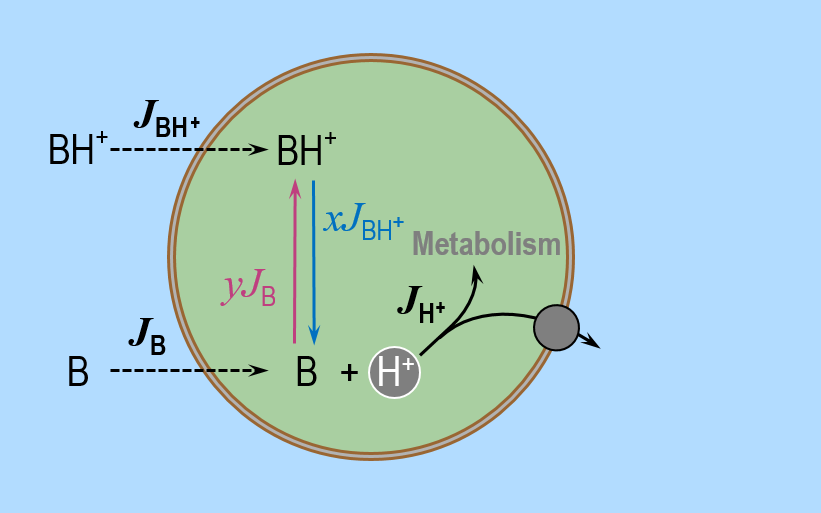Figure 5:Cartoon illustrating the main assumptions in the BDW model of permeating uncharged weak base B\mathrm{B}B and its conjugate anionic weak acid BH+\mathrm{BH^+}BH+. The BDW model consists of two time-dependent ODEs. The first one describes the time-course of the intracellular concentration of total weak base [TB]i\mathrm{[TB]_i}[TB]i​, and the second one describes the time-course of [H+]i\mathrm{[H^+]_i}[H+]i​. BDW assumed that [TB]i\mathrm{[TB]_i}[TB]i​ changes in time because of the transmembrane fluxes of HA\mathrm{HA}HA (JBJ_\mathrm{B}JB​) — modelled according to Fick’s first law of diffusion — and BH+\mathrm{BH^+}BH+ (JBH+J_\mathrm{BH^+}JBH+​) — modelled according to the Goldman, Hodgkin, Katz (GHK) equation. According to BDW, the time rate of change of [H+]i\mathrm{[H^+]_i}[H+]i​ depends on the net rate dQ/dt\mathrm{d}Q/\mathrm{d}tdQ/dt at which acids are added into the cytosol. BDW assumed that dQ/dt\mathrm{d}Q/\mathrm{d}tdQ/dt depends on (i) the release of H+\mathrm{H^+}H+ by some fraction xxx of the entering BH+\mathrm{BH^+}BH+ (i.e., xJBH+xJ_\mathrm{BH^+}xJBH+​), (ii) the consumption of H+\mathrm{H^+}H+ by some fraction yyy of the entering B\mathrm{B}B (i.e., yJByJ_\mathrm{B}yJB​), and (iii) the additional rate of intracellular H+\mathrm{H^+}H+ consumption via metabolism or active acid extrusion (JH+J_\mathrm{H^+}JH+​).

Assuming, as in Figure 5, that $\mathrm{[TB]_i}$ only can change due to the transmembrane fluxes of $\mathrm{B}$ ($J_\mathrm{B}$) and $\mathrm{BH^+}$ ($J_{\mathrm{BH^+}}$), the time rate of change of $\mathrm{[TB]_i]}$ — analogous to (11) above — is

$\dfrac{\mathrm{d[TB]_i}}{\mathrm{d}t}=\rho\left(J_\mathrm{B}+J_\mathrm{BH^+}\right),$

where $\rho$ ($\mathrm{m^{-1}}$) is again the area-to-volume ratio for the cell. The equation

$J_\mathrm{B}=P_\mathrm{B}\bigg(\mathrm{[B]_o}-\mathrm{[B]_i}\bigg),$

is an integrated form of Fick’s first law of diffusion that describes the net passive flux of B, and

$J_\mathrm{BH^+}=P_\mathrm{BH^+}\left(\dfrac{V_\mathrm{m}F}{RT}\right)\left(\dfrac{\mathrm{[BH^+]_o}-\epsilon '\mathrm{[BH^+]_i}}{\epsilon '-1}\right),$

describes the net passive influx of $\mathrm{BH^+}$ according to the GHK equation. In the previous two equations, $P_\mathrm{B}$ ($\mathrm{m\cdot s^{-1}}$) is the membrane permeability to the uncharged weak base $\mathrm{B}$, $P_\mathrm{{BH^+}}$ ($\mathrm{m\cdot s^{-1}}$) is the membrane permeability to the charged conjugate weak acid $\mathrm{BH^+}$, and $\epsilon '$ is a shorthand for $e^{{V_\mathrm{m}F}/{RT}}$. (33) is the first of two ODEs of the BDW model for the buffer pair $\mathrm{B}/\mathrm{BH^+}$.

The second equation of the BDW model for a weak base — analogous to (27) above — is

$\dfrac{\mathrm{d[H^+]_i}}{\mathrm{d}t}=\left(\dfrac{2.303\mathrm{[H^+]_i}}{\beta}\right) \rho\bigg((1-\alpha)J_\mathrm{BH^+}-\alpha J_\mathrm{B}-J_\mathrm{H^+}\bigg),$

where $J_\mathrm{H+}$ is the same as in (18) and

$\alpha=\dfrac{\mathrm{[BH^+]_i}}{\mathrm{[BH^+]_i}+\mathrm{[B]_i}}=\dfrac{\mathrm{[H^+]_i}}{\mathrm{[H^+]_i}+K_\mathrm{BH^+}},$

and

$1-\alpha=\dfrac{\mathrm{[B]_i}}{\mathrm{[BH^+]_i}+\mathrm{[B]_i}}=\dfrac{K_\mathrm{BH^+}}{\mathrm{[H^+]_i}+K_\mathrm{BH^+}}.$

Substituting for $\alpha$, $[\mathrm{BH^+]_i} = \alpha [\mathrm{TB]_i}, [\mathrm{B]_i} = (1-\alpha) [\mathrm{TB]_i}$, $J_\mathrm{BH^+}$ , $J_\mathrm{B}$ in (33) and (36), we obtain the two ODEs of the BDW model in terms of $[\mathrm{TB]_i}$ and $\mathrm{[H^+]_i}$

$\dfrac{\mathrm{d[TB]_i}}{\mathrm{d}t}=\rho\left(J_\mathrm{B}+J_\mathrm{BH^+} \right),$
$\dfrac{\mathrm{d[H^+]_i}}{\mathrm{d}t}=\dfrac{2.303\mathrm{[H^+]_i}}{\beta}\rho\left(\left(\dfrac{K_\mathrm{BH^+}}{\mathrm{[H^+]_i}+K_\mathrm{BH^+}}\right)J_\mathrm{BH^+}-\left(\dfrac{\mathrm{[H^+]_i}}{\mathrm{[H^+]_i}+K_\mathrm{BH^+}}\right)J_\mathrm{B}-J_\mathrm{H^+} \right),$

where

$J_\mathrm{BH^+}=P_\mathrm{BH^+}\left(\dfrac{V_\mathrm{m}F}{RT}\right)\left(\dfrac{\mathrm{[BH^+]_o}-\dfrac{\mathrm{[H^+]_i}}{\mathrm{[H^+]_i}+K_\mathrm{BH^+}}\mathrm{[TB]_i} \epsilon '}{\epsilon '-1}\right),$
$J_\mathrm{B}=P_\mathrm{B}\left( \mathrm{[B]_o}-\dfrac{K_\mathrm{BH^+}}{\mathrm{[H^+]_i}+K_\mathrm{BH^+}}\mathrm{[TB]_i} \right),$

and $J_\mathrm{H^+}$ is given by (18).

Numerically integrating the above two equations yields the time courses of $[\mathrm{TB]_i}$ and $\mathrm{[H^+]_i}$, from which we can compute the time-courses of $[\mathrm{BH^+]_i}$ and $[\mathrm{B]_i}$ from

$\mathrm{[BH^+]_i}=\alpha\mathrm{[TB]_i},$
$\mathrm{[B]_i}=(1-\alpha)\mathrm{[TB]_i},$

where

$\alpha=\dfrac{\mathrm{[H^+]_i}}{\mathrm{[H^+]_i}+K_\mathrm{BH^+}}.$
##### Simulation for $\mathrm{NH_3}/\mathrm{NH_4^+}$ experiments.¶

BDW employed (39) and (40) to simulate the experiments in which they exposed a squid giant axon to equilibrated $\mathrm{NH_3}/\mathrm{NH_4^+}$. Their simulation protocol was a step change in extracellular $\mathrm{NH_4Cl}$ from $0$ to $9$ $\mathrm{mM}$ (that is, a step change in $\mathrm{[NH_4^+]_o}$ from $0$ to $8.86$ $\mathrm{mM}$, and in $\mathrm{[NH_3]_i}$ from $0$ to $0.14$ $\mathrm{mM}$) applied for $1500~\mathrm{s}$ ($25~\mathrm{min}$) at constant $\mathrm{pH_o}=7.70$.

Table 1 and Table 3 report the parameter values used by BDW. Note that in the $\mathrm{NH_3}/\mathrm{NH_4^+}$ simulations, $k$ is always zero, that is, $J_\mathrm{H^+}$ does not affect these processes.